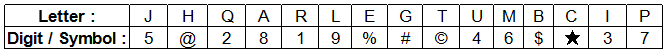## IBPS Reasoning Test 23

Instructions

In each question below is given a group of letters followed by four combinations of digits/symbols numbered a, b, c and d. You have to find out which of the combinations correctly represents the group of letters based on the following coding system and mark the number of that combination as the answer. If none of the four combinations correctly represents the group of letters, mark e: i.e. ‘None of these’ as the answer. SymbolConditions :
(i) If both the first and the last letters of the group are vowels, their codes are to be interchanged.
(ii) If the first letter is a consonant and the last letter is a vowel, both are to be coded by the code for the consonant.

Question 1

JQGALI

Question 2

EBHRMT

Question 3

IGCHRE

Question 4

BQRLHA

Question 5

QRLGHM